# Python图像处理PIL各模块详细介绍图像处理 同时被 2 个专栏收录10 篇文章 11 订阅

# Image模块

Image模块是在Python PIL图像处理中常见的模块，对图像进行基础操作的功能基本都包含于此模块内。如opensaveconvershow…等功能。

## open类

Image.open(file) ⇒ image
Image.open(file, mode) ⇒ image

    @zhangziju
from PIL import Image             ##调用库
im = Image.open("E:\mywife.jpg")  ##文件存在的路径
im.show()## Save类

im.save(outfile,options…)
im.save(outfile, format, options…)

@zhangziju
from PIL import Image
im = Image.open("E:\mywife.jpg")
print(im)
im.save("E:\mywife.png")     ## 将"E:\mywife.jpg"保存为"E:\mywife.png"
im = Image.open("E:\mywife.png")  ##打开新的png图片
print(im.format, im.size, im.mode)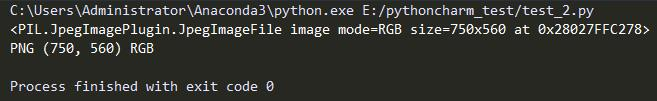## format类

im.format ⇒ string or None

@zhangziju
from PIL import Image
im = Image.open("E:\mywife.jpg")
print(im.format) ## 打印出格式信息
im.show()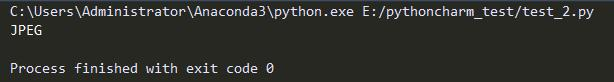## Mode类

im.mode ⇒ string

modes描述
11位像素，黑和白，存成8位的像素
L8位像素，黑白
P8位像素，使用调色板映射到任何其他模式
RGB3× 8位像素，真彩
RGBA4×8位像素，真彩+透明通道
CMYK4×8位像素，颜色隔离
YCbCr3×8位像素，彩色视频格式
I32位整型像素
F32位浮点型像素
@zhangziju
from PIL import Image
im = Image.open("E:\mywife.jpg")
print(im.mode) ## 打印出模式信息
im.show()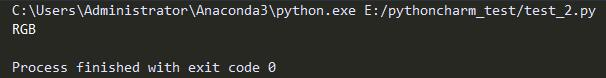## convert类

im.convert(mode)⇒ image

L = R * 299/1000 + G * 587/1000 + B * 114/1000

from PIL import Image
im = Image.open("E:\mywife.jpg")
new_im = im.convert('P')
print(new_im.mode)
new_im.show()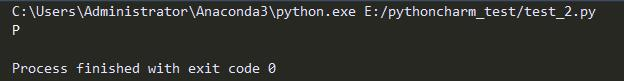im.convert(“P”,**options) ⇒ image

Dither=. 控制颜色抖动。默认是FLOYDSTEINBERG，与邻近的像素一起承担错误。不使能该功能，则赋值为NONE。

im.convert(mode,matrix) ⇒ image


from PIL import Image
im = Image.open("E:\mywife.jpg")
print(im.mode)
rgb2xyz = (0.412453,0.357580, 0.180423, 0,
0.212671,0.715160, 0.072169, 0,
0.019334,0.119193, 0.950227, 0 )
new_im = im.convert("L", rgb2xyz)
print(new_im.mode)
new_im.show()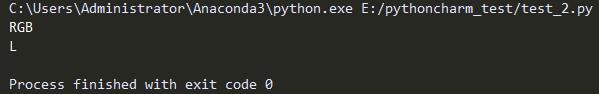## Size类

im.size ⇒ (width, height)

@zhangziju
from PIL import Image
im = Image.open("E:\mywife.jpg")
print(im.size) ## 打印出尺寸信息
im.show()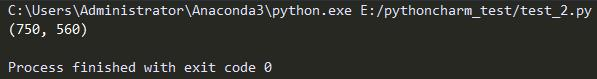## Palette类

im.palette ⇒ palette or None

@zhangziju
from PIL import Image
im = Image.open("E:\mywife.jpg")
print(im.palette)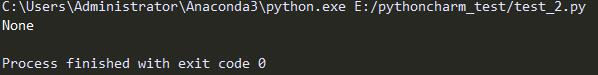@zhangziju
from PIL import Image
im = Image.open("E:\mywife.jpg")
new_im = im.convert('P')
print(new_im.mode)
print(new_im.palette)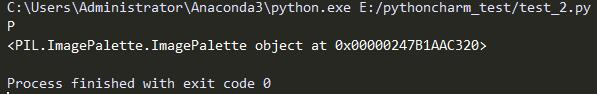## Info类

im.info ⇒ dictionary

@zhangziju
from PIL import Image
im = Image.open("E:\mywife.jpg")
print(im.info)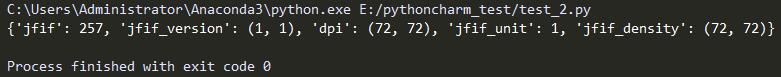## new类

Image.new(mode,size) ⇒ image
Image.new(mode, size,color) ⇒ image

@zhangziju
from PIL import Image
im = Image.open("E:\mywife.jpg")
n_im= Image.new("RGB", (128, 128), "#FF0000")
n_im.show()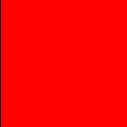@zhangziju
from PIL import Image
im = Image.open("E:\mywife.jpg")
n_im= Image.new("RGB", (128, 128))
n_im.show()@zhangziju
from PIL import Image
im = Image.open("E:\mywife.jpg")
n_im= Image.new("RGB", (128, 128),"green")
n_im.show()## Copy类

im.copy() ⇒ image


from PIL import Image
im = Image.open("E:\mywife.jpg")
im_copy = im.copy()

## Crop类

im.crop(box) ⇒ image@zhangziju
from PIL import Image
im = Image.open("E:\mywife.jpg")
box = (300, 100, 700, 700)              ##确定拷贝区域大小
region = im.crop(box)                   ##将im表示的图片对象拷贝到region中，大小为box
region.show()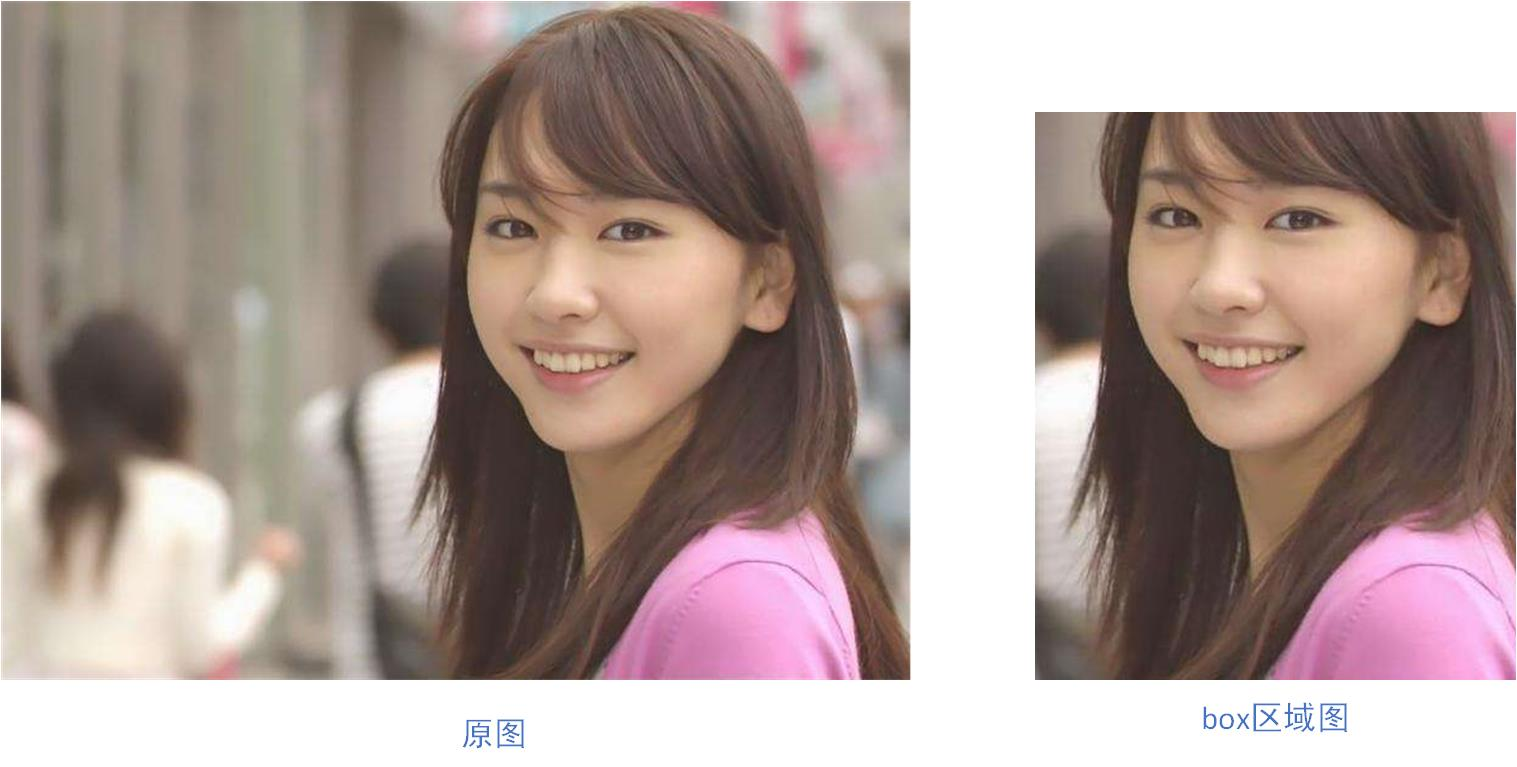## Paste类

im.paste(image,box)

@zhangziju
from PIL import Image
im = Image.open("E:\mywife.jpg")
box=[0,0,100,100]
im_crop = im.crop(box)
print(im_crop.size,im_crop.mode)
im.paste(im_crop, (100,100))             ##(100,100,0,0)
im.paste(im_crop, (400,400,500,500))
im.show()## Filter类

im.filter(filter) ⇒ image

@zhangziju
from PIL import Image
from PIL import ImageFilter                         ## 调取ImageFilter
imgF = Image.open("E:\mywife.jpg")
bluF = imgF.filter(ImageFilter.BLUR)                ##均值滤波
conF = imgF.filter(ImageFilter.CONTOUR)             ##找轮廓
edgeF = imgF.filter(ImageFilter.FIND_EDGES)         ##边缘检测
imgF.show()
bluF.show()
conF.show()
edgeF.show()## Blend类

Image.blend(image1,image2, alpha) ⇒ image

@zhangziju
from PIL import Image
im1 = Image.open("E:\mywife.jpg")
im2 = Image.open("E:\mywife2.jpg")
print(im1.mode,im1.size)
print(im2.mode,im2.size)
im = Image.blend(im1, im2, 0.2)
im.show()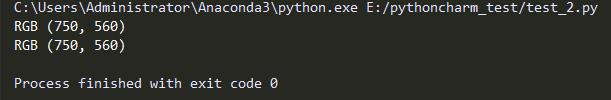im1和im2按照第一张80%的透明度，第二张20%的透明度，合成为一张。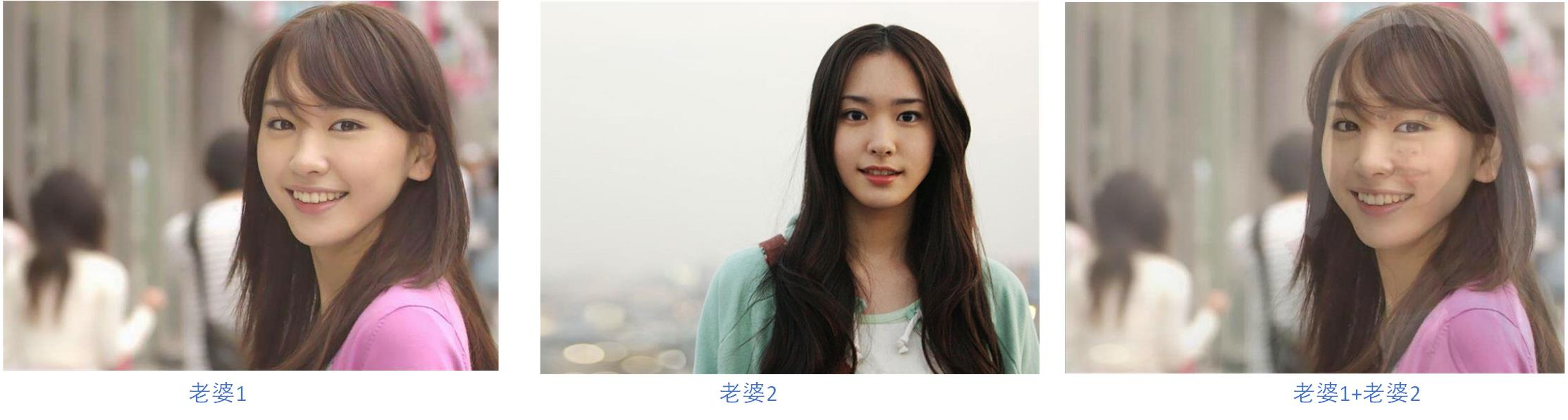## Split

im.split() ⇒ sequence

from PIL import Image
im = Image.open("E:\mywife.jpg")
r,g,b = im.split()
print(r.mode)
print(r.size)
print(im.size)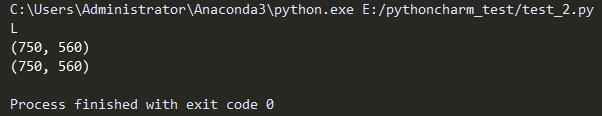## Composite类

@zhangziju
from PIL import Image
im1 = Image.open("E:\mywife.jpg")
im2 = Image.open("E:\mywife2.jpg")
r,g,b = im1.split()             ##分离出r，g，b
print(b.mode)
print(im1.mode,im1.size)
print(im2.mode,im2.size)
im = Image.composite(im1,im2,b)
im.show()

b.mode为”L”，两图尺寸一致。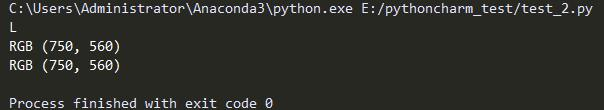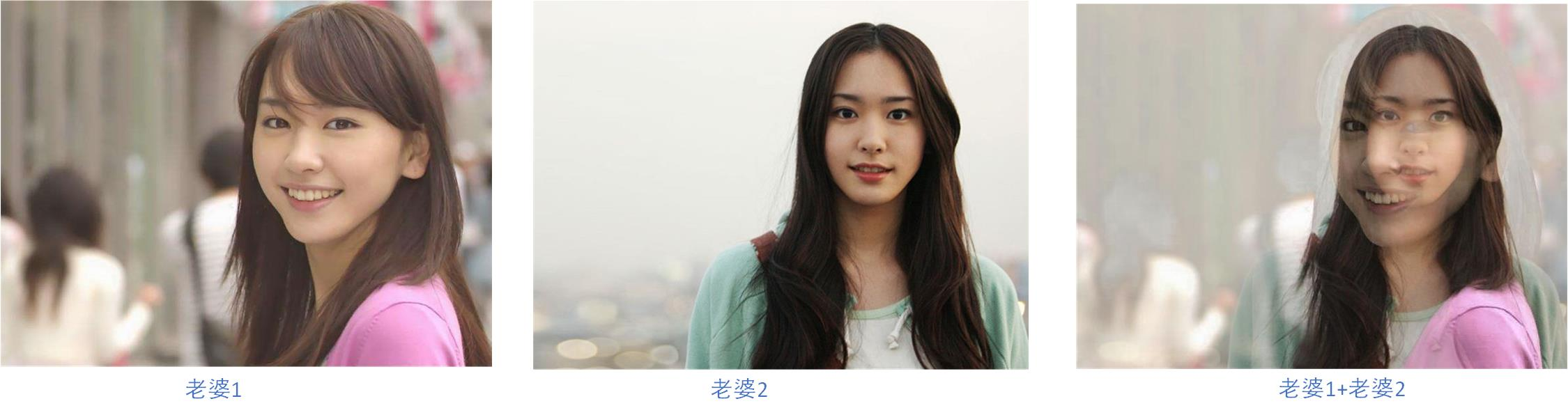## Eval类

Image.eval(image,function) ⇒ image

@zhangziju
from PIL import Image
im = Image.open("E:\mywife.jpg")
def fun01(x):
return x*0.3
def fun02(y):
return y*2.0
im1_eval = Image.eval(im, fun01)
im2_eval = Image.eval(im, fun02)
im1_eval.show()
im2_eval.show()## Merge类

Image.merge(mode,bands) ⇒ image

len(ImageMode.getmode(mode).bands)= len(bands)

@zhangziju
from PIL import Image
im1 = Image.open("E:\mywife.jpg")
im2 = Image.open("E:\mywife2.jpg")
r1,g1,b1 = im1.split()
r2,g2,b2 = im2.split()
print(r1.mode,r1.size,g1.mode,g1.size)
print(r2.mode,r2.size,g2.mode,g2.size)
new_im=[r1,g2,b2]
print(len(new_im))
im_merge = Image.merge("RGB",new_im)
im_merge.show()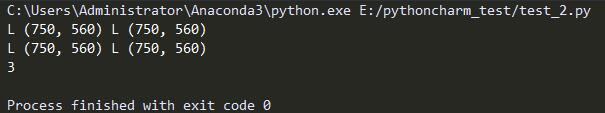merge操作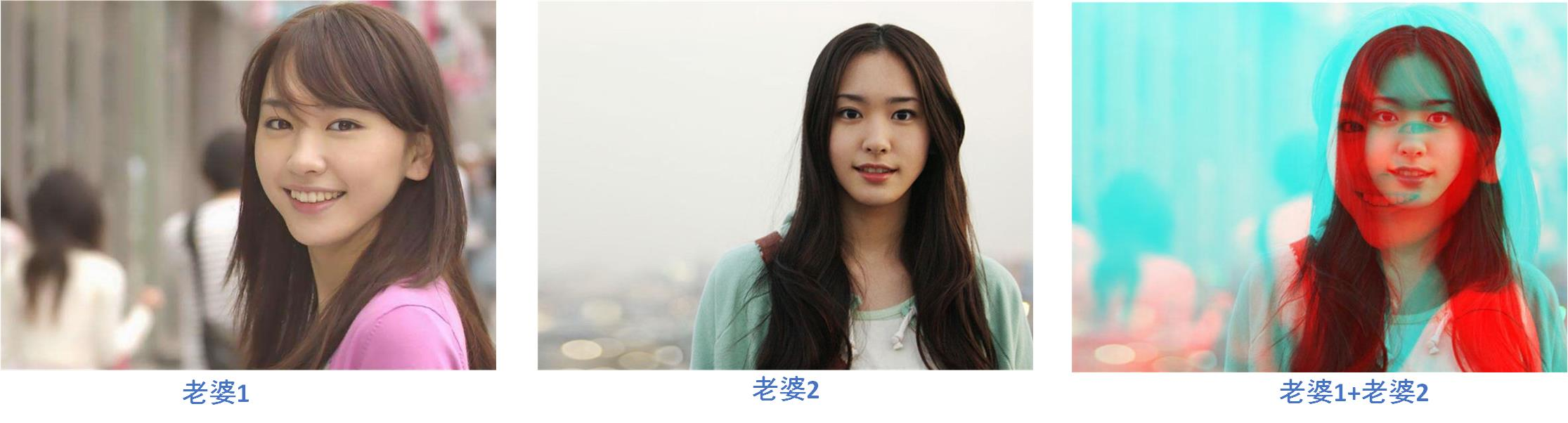## Draft类

im.draft(mode,size)

@zhangziju
from PIL import Image
im = Image.open("E:\mywife.jpg")
print(im.size,im.mode)
new_im = im.draft("L", (200,200))
print(new_im.size,new_im.mode)
new_im.show()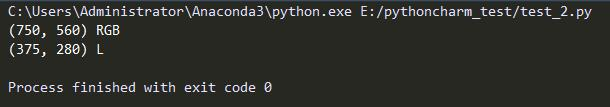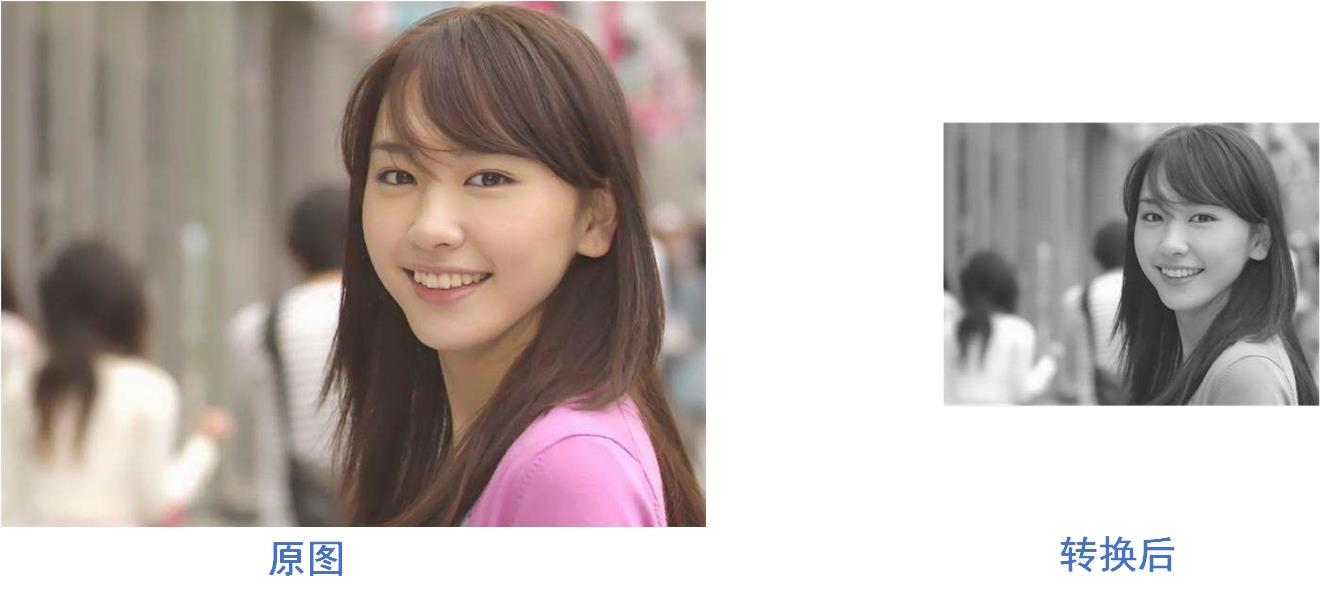## Getbands类

im.getbands()⇒ tuple of strings

@zhangziju
from PIL import Image
im = Image.open("E:\mywife.jpg")
print(im.getbands())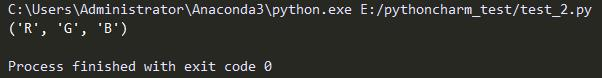## Getbbox类

im.getbbox() ⇒ 4-tuple or None

@zhangziju
from PIL import Image
im = Image.open("E:\mywife.jpg")
print(im.getbbox())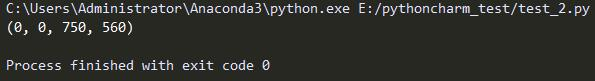## Getdata类

im.getdata() ⇒ sequence

@zhangziju
from PIL import Image
im = Image.open("E:\mywife.jpg")
sequ = im.getdata()
sequ0 = list(sequ)
print(sequ0)
print(sequ0)
print(sequ0)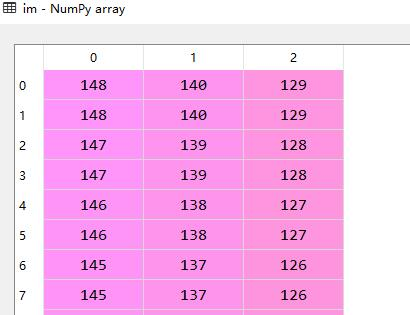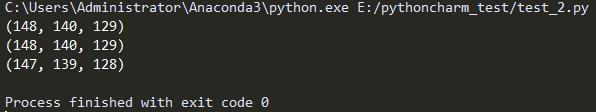## Getextrema类

im.getextrema() ⇒ 2-tuple

@zhangziju
from PIL import Image
im = Image.open("E:\mywife.jpg")
print(im.getextrema())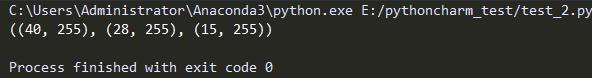# Getpixel类

im.getpixel(xy) ⇒ value or tuple

@zahngziju
from PIL import Image
im = Image.open("E:\mywife.jpg")
print(im.getpixel((0,0)))
print(im.getpixel((4,0)))
r,g,b = im.split()
print(b.getpixel((11,8)))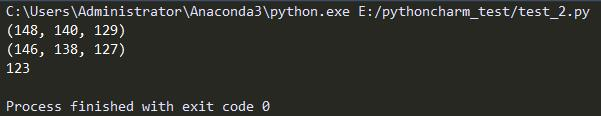## Histogram类

im.histogram()⇒ list

@zhangziju
from PIL import Image
im = Image.open("E:\mywife.jpg")
imhis = im.histogram()
print(len(imhis))
print(imhis)
print(imhis)
print(imhis)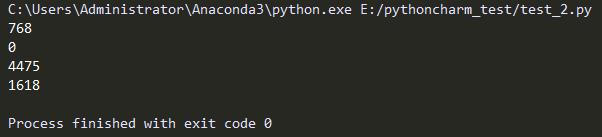@zhangziju
from PIL import Image
im = Image.open("E:\mywife.jpg")
r,g,b = im.split()
imhis = im.histogram()
print(r.mode)
print(len(imhis))
print(imhis)
print(imhis)
print(imhis)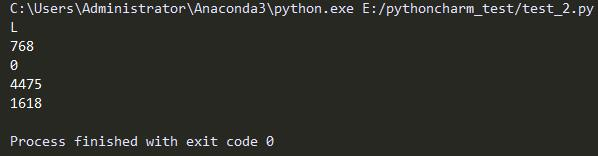print pix[x, y]
pix[x, y] =value

@zhangziju
from PIL import Image
im = Image.open("E:\mywife.jpg")
print(pix[0,2])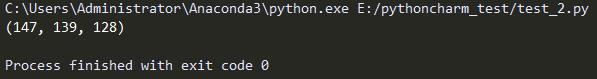im.paste(colour,box)

@zhangziju
from PIL import Image
im = Image.open("E:\mywife.jpg")
im.paste((256,256,0),(0,0,100,100))  ##(256,256,0)表示黄色
im.show()@zhangziju
from PIL import Image
im = Image.open("E:\mywife.jpg")
im.paste("blue",(0,0,100,100))   ##或者“blue”
im.show()@zhangziju
from PIL import Image
im = Image.open("E:\mywife.jpg")
box=[300,300,400,400]
im_crop =im.crop(box)
r,g,b =im_crop.split()
im.paste(im_crop, (200,200,300,300), r)
im.show()## Putdata类

im.putdata(data)
im.putdata(data, scale, offset)

pixel = value*scale + offset

@zhangziju
from PIL import Image
im = Image.open("E:\mywife.jpg")
r, g, b = im.split()
print(
r.getpixel((0, 0)),
r.getpixel((1, 0)),
r.getpixel((2, 0)),
r.getpixel((3, 0)),
r.putdata([1, 2, 3, 4]),
r.getpixel((0, 0)),
r.getpixel((1, 0)),
r.getpixel((2, 0)),
r.getpixel((3, 0)),
)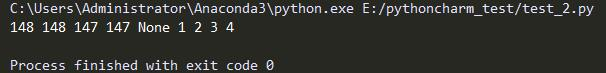## Resize类

im.resize(size) ⇒ image
im.resize(size, filter) ⇒ image

@zhangziju
from PIL import Image
im = Image.open("E:\mywife.jpg")
region = im.resize((400, 400))     ##重新设定大小
region.show()## Rotate类

im.rotate(angle) ⇒ image
im.rotate(angle,filter=NEAREST, expand=0) ⇒ image

@zhangziju
from PIL import Image
im = Image.open("E:\mywife.jpg")
im_45 = im.rotate(45)
im_30 = im.rotate(30, Image.NEAREST,1)
print(im_45.size,im_30.size)
im_45.show()
im_30.show()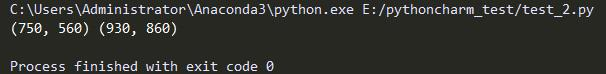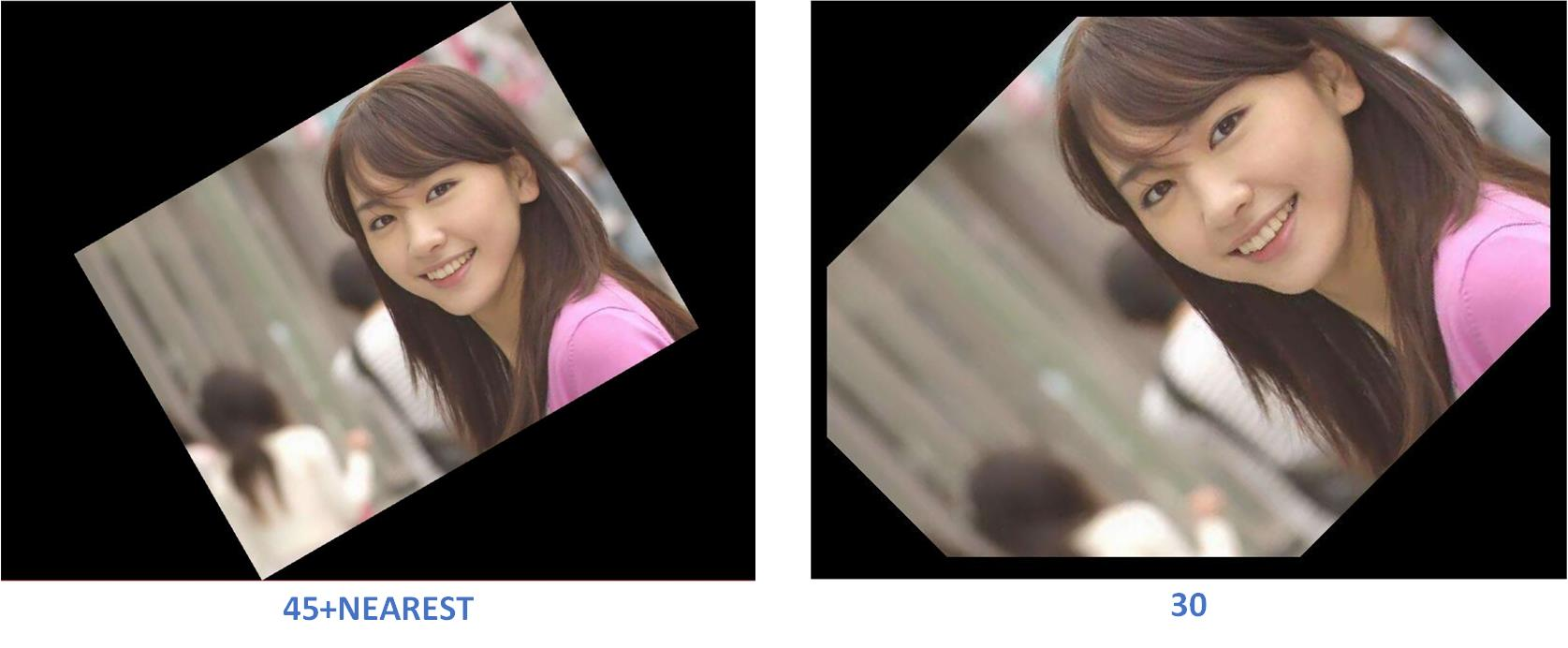## Seek类

im.seek(frame)

@zhangziju
from PIL import Image
im_gif = Image.open("E:\mywife.gif")
print(im_gif.mode)
im_gif.show()    ##第0帧
im_gif.seek(3)
im_gif.show()
im_gif.seek(9)
im_gif.show()## Tell类

im.tell() ⇒ integer

@zhangziju
from PIL import Image
im_gif = Image.open("E:\mywife.gif")
print(im_gif.tell())
im_gif.seek(8)
print(im_gif.tell())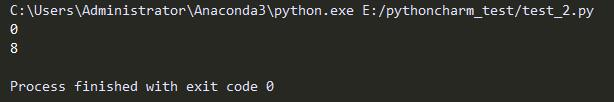## Thumbnail类

im.thumbnail(size)
im.thumbnail(size, filter)

@zhangziju
from PIL import Image
im = Image.open("E:\mywife.jpg")
im.thumbnail((100,100))

## Transform类

im.transform(size,method, data) ⇒ image
im.transform(size, method, data, filter) ⇒ image

@zhangziju
from PIL import Image
im = Image.open("E:\mywife.jpg")
print(im.size)
imtra = im.transform((200, 200), Image.EXTENT, (0, 0, 500, 500))
print(imtra.size)
imtra.show()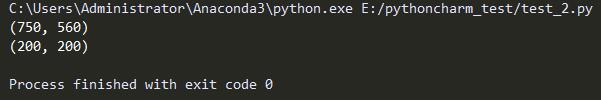im.transform(size,EXTENT, data) ⇒ image
im.transform(size, EXTENT, data, filter) ⇒ image

im.transform(size, AFFINE, data) ⇒ image
im.transform(size, AFFINE,data, filter) ⇒ image

@zhangziju
from PIL import Image
im = Image.open("E:\mywife.jpg")
print(im.size)
imtra = im.transform((200, 200), Image.AFFINE, (1,2,3,2,1,4))
print(imtra.size)
imtra.show()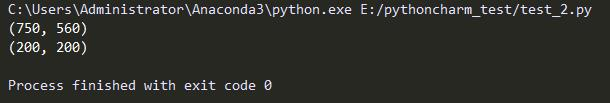im.transform(size, QUAD, data, filter) ⇒ image

@zhangziju
from PIL import Image
im = Image.open("E:\mywife.jpg")
print(im.size)
imtra = im.transform((200, 200), Image.QUAD, (0,0,0,500,600,500,600,0))
print(imtra.size)
imtra.show()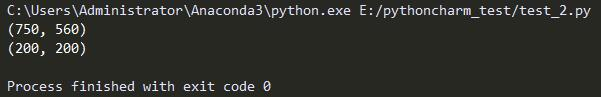im.transform(size,PERSPECTIVE, data) ⇒ image
im.transform(size, PERSPECTIVE, data, filter) ⇒ image

@zhangziju
from PIL import Image
im = Image.open("E:\mywife.jpg")
print(im.size)
imtra = im.transform((200, 200), Image.PERSPECTIVE, (1,2,3,2,1,6,1,2))
print(imtra.size)
imtra.show()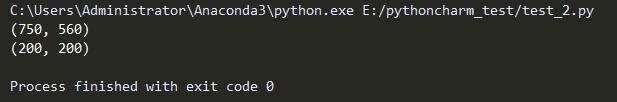wocao!!!gakki不见了！！！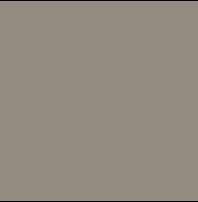## Transpose类

im.transpose(method)⇒ image

@zhangziju
from PIL import Image
im = Image.open("E:\mywife.jpg")
im.rotate(45)                             #逆时针旋转 45 度角。
im.transpose(Image.FLIP_LEFT_RIGHT)       #左右对换。
im.transpose(Image.FLIP_TOP_BOTTOM)       #上下对换。
im.transpose(Image.ROTATE_90)             #旋转 90 度角。
im.transpose(Image.ROTATE_180)            #旋转 180 度角。
im.transpose(Image.ROTATE_270)            #旋转 270 度角。
11-0617万+11-071万+
03-054397
12-091万+
02-275313
02-212万+
03-263948
01-173万+
02-111万+
05-034326
03-1020万+
12-07415
03-251658
03-304万+
04-021万+
05-086万+
05-081万+点击重新获取扫码支付余额充值